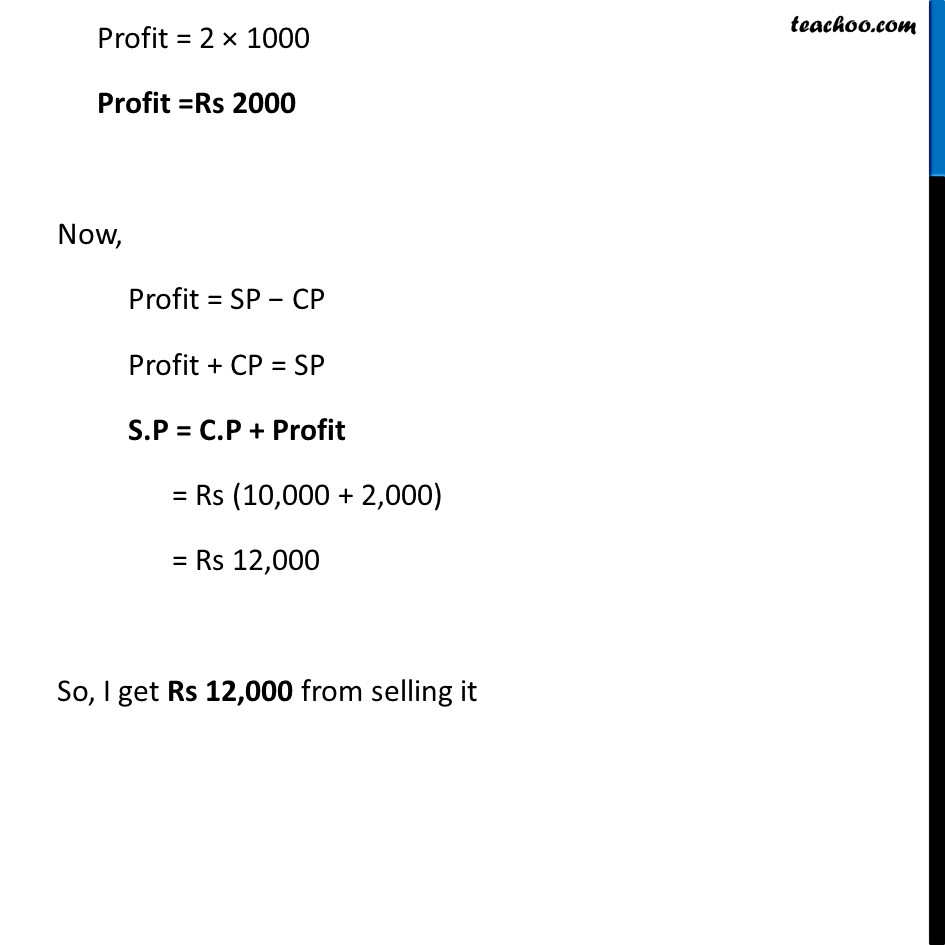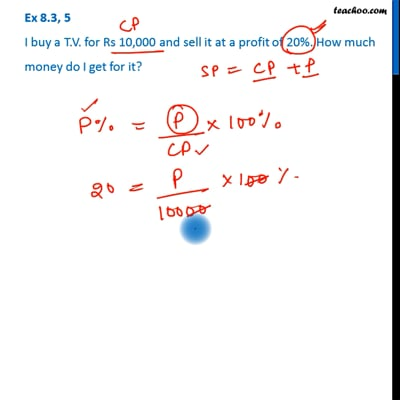Ex 7.2

Chapter 7 Class 7 Comparing Quantities
Serial order wiseThis video is only available for Teachoo black users

Learn in your speed, with individual attention - Teachoo Maths 1-on-1 Class

### Transcript

Ex 7.2, 5 I buy a T.V. for Rs 10,000 and sell it at a profit of 20%. How much money do I get for it? C.P = Rs 10,000 S.P = ? Profit percentage = 20% 𝑃𝑟𝑜𝑓𝑖𝑡/(𝐶.𝑃) × 100 = 20 𝑷𝒓𝒐𝒇𝒊𝒕/𝟏𝟎𝟎𝟎𝟎 × 100 = 20 Profit = 20/100 × 10000 Profit = 2/10 × 10000 Profit = 2 × 1000 Profit =Rs 2000 Now, Profit = SP − CP Profit + CP = SP S.P = C.P + Profit = Rs (10,000 + 2,000) = Rs 12,000 So, I get Rs 12,000 from selling it